R packages:

# Install necessary packages
list_packages <- c("forecast", "readxl", "stargazer", "fpp",
"fpp2", "scales", "quantmod", "urca",
"vars", "tseries", "ggplot2", "dplyr")
new_packages <- list_packages[!(list_packages %in% installed.packages()[,"Package"])]
if(length(new_packages)) install.packages(new_packages)

lapply(list_packages, require, character.only = TRUE)

# 1 ARMA Models

## 1.1 ARMA(1,1)

ARMA(1,1) can be written as any of the following forms. \begin{align*} \tilde{Z}_t&=\phi_1 \tilde{Z}_{t-1} + a_t - \theta_1 a_{t-1} \end{align*}

ACF and PACF of ARMA(1,1)

n=200000
ts1=arima.sim(n = n, list(ar = 0.6, ma = 0.7), sd = 1)
par(mfrow=c(1,2))
acf(ts1,ylim=c(-1,1),lag.max=10)
pacf(ts1,ylim=c(-1,1),lag.max=10)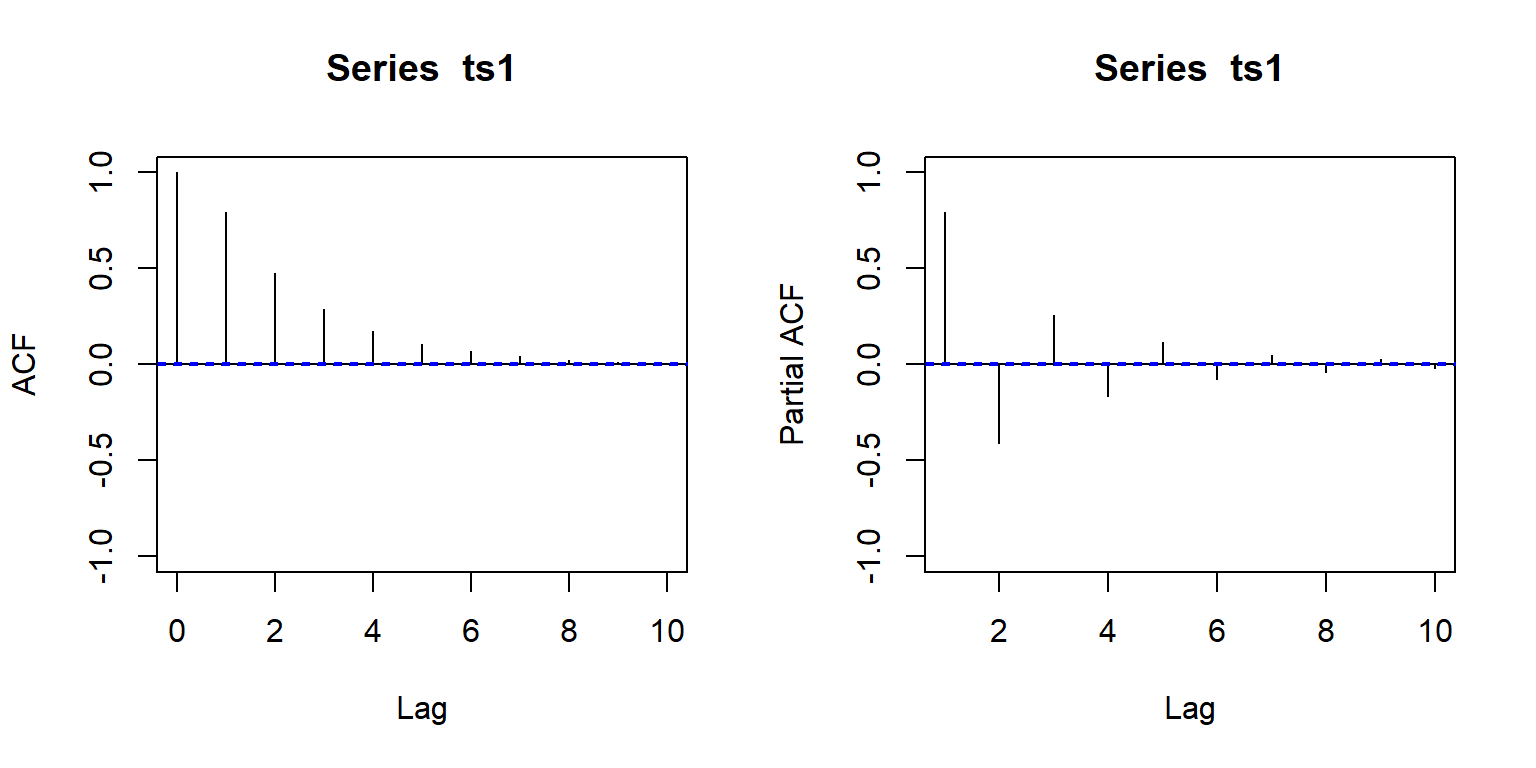## 1.2 ARMA(p,q)

ARMA(p,q) can be written as any of the following forms. \begin{align*} \phi(B)\tilde{Z}_t&=\theta(B)a_t\\ (1 - \phi_1 B - ... -\phi_p B^p)\tilde{Z}_t&=(1 - \theta_1 B ... - \theta_q B^q)a_t\\ \tilde{Z}_t&= \phi_1 \tilde{Z}_{t-1} + ... +\phi_p \tilde{Z}_{t-p} + a_t - \theta_1 a_{t-1} ... - \theta_q a_{t-q}\\ Z_t &= c + \phi_1 Z_{t-1} + ... +\phi_p Z_{t-p} + a_t - \theta_1 a_{t-1} ... - \theta_q a_{t-q} \end{align*} where $$c=\mu(1-\phi_1 ... - \phi_p)$$.

ACF and PACF of ARMA(p,q)

n=200000
ts1=arima.sim(n = n, list(ar = c(0.6,0.1,-0.3), ma = c(0.5,0.3)), sd = 1)
par(mfrow=c(1,2))
acf(ts1,ylim=c(-1,1),lag.max=10)
pacf(ts1,ylim=c(-1,1),lag.max=10)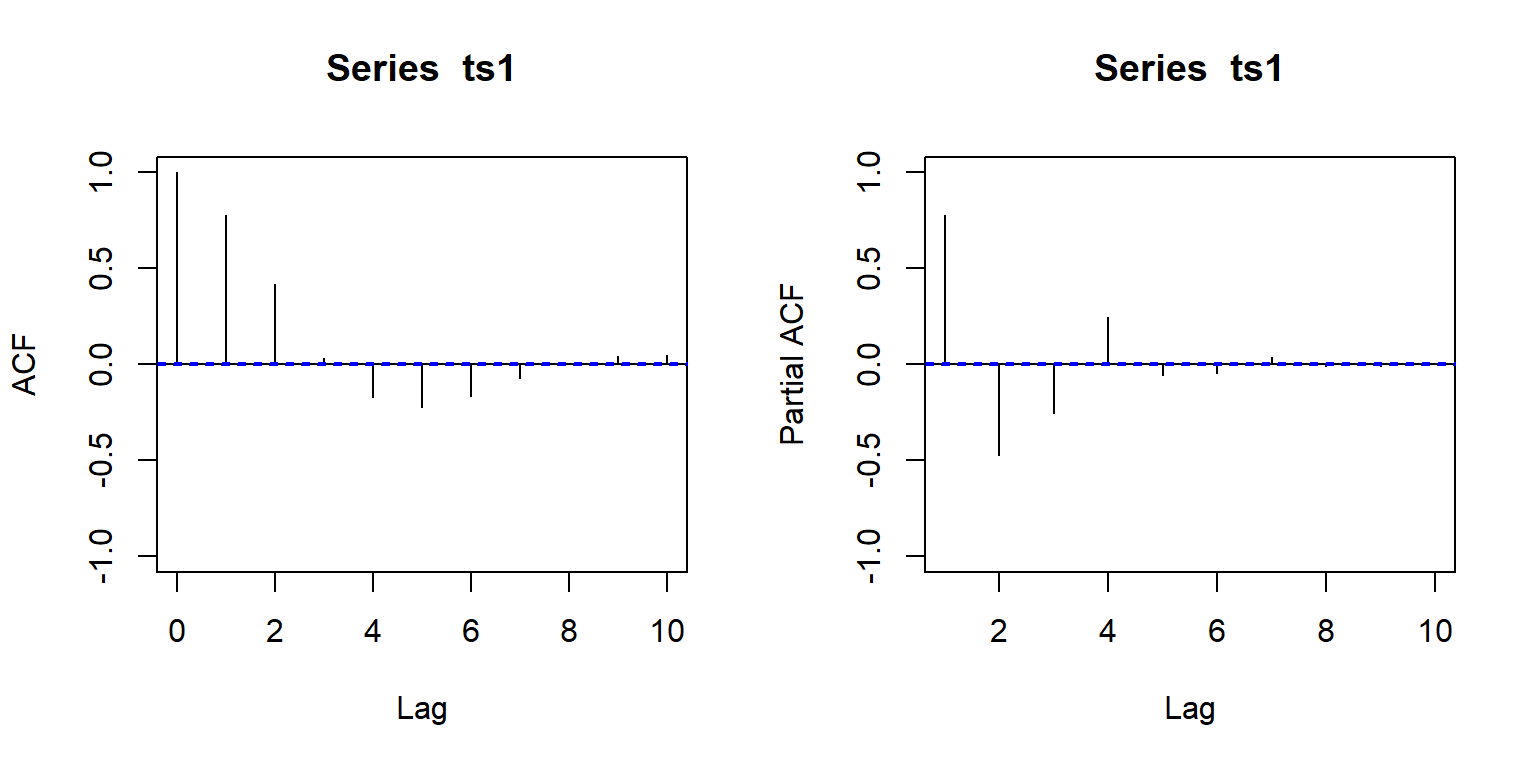# 2 ARIMA Models

Some time series encountered in real world are not stationary. For example, they have no fixed mean. Many have a behavior called homogeneous nonstationarity, i.e., the levels of time series are different at different time periods.

Homogeneous nonstationarity is nonstationarity which can be attributed solely to difference in mean or trend (not due to difference in variance.)

n=2000
ts1=arima.sim(n = n, list(ar = 0.5, ma = 0.3), sd = 1)
ts1=ts1+c(rep(0,500),rep(3,1000),rep(-2,500))
ts2=arima.sim(n = n, list(ar = 0.5, ma = 0.3), sd = 1)
ts2=ts2+c(seq(0,3,length.out=500),seq(3,-2,length.out=1000),seq(-2,0,length.out=500))
par(mfrow=c(2,1))
plot(ts1,main="different means")
plot(ts2,main="different trends")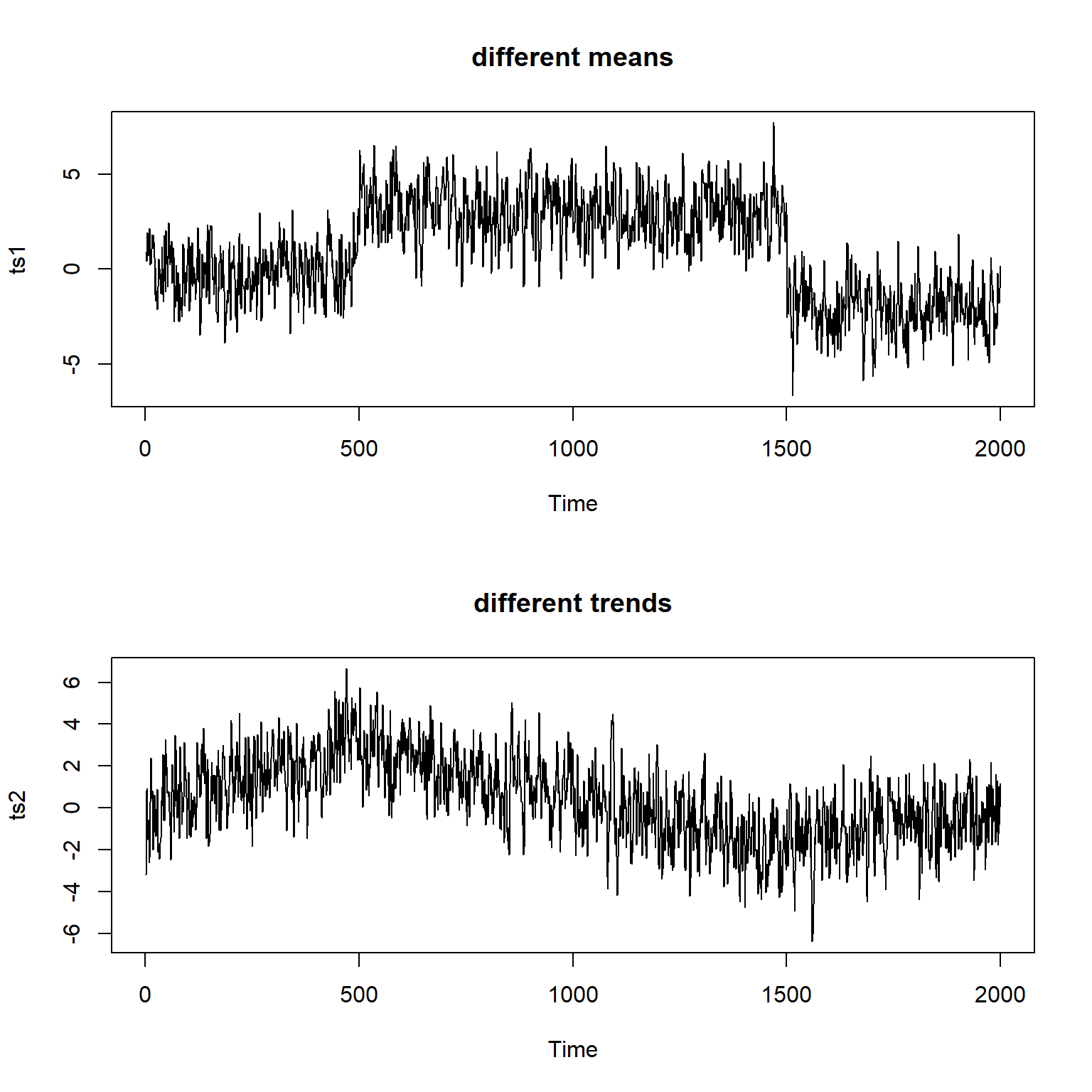These kinds of time series models are well represented by ARIMA model, autoregressive integrated moving average model.

\begin{align*} (1-\phi_1 B - ... - \phi_p B^p)(1-B)^d\tilde{Z}_t&=(1-\theta_1 B - ... - \theta_q B^q)a_t\\ \phi(B)(1-B)^d\tilde{Z}_t&=\theta(B)a_t\\ \phi(B)\nabla^d\tilde{Z}_t&=\theta(B)a_t\\ \varphi(B)\tilde{Z}_t&=\theta(B)a_t\\ \end{align*} where $$\nabla = 1-B$$ is the difference operator. This is called ARIMA of order (p,d,q) where $$p$$ is the AR order, $$q$$ is the MA order, $$d$$ is difference order.

That is, at least one of the roots of $$\varphi(B)=0$$ lies on the unit circle.

For such a time series model, we assume that there exists a $$d$$ such that $$\nabla^d \tilde{Z}_t$$ is a stationary ARMA process.

For example 1 above, we could use $$d=1$$, i.e., first order difference.

For example 2 above, we could use $$d=2$$, i.e., second order difference.

Here is another example, suppose $$\tilde{Z}_t$$ is ARIMA(1,2,3), then let $$W_t = \nabla^2 \tilde{Z}_t$$ is ARMA(1,3).

Taking difference is essentially removing homogeneous nonstationarity.

## 2.1 ACF and PACF of ARIMA

The ACF of a nonstationary ARIMA fails to die out rapidly. If we observe that ACFs are significantly different from zero for many $$k$$, we should inspect the 1st order difference series, $$W_t=\nabla \tilde{Z}_t$$, the 2nd order difference series, $$W_t=\nabla^2 \tilde{Z}_t$$, and so on, until ACFs dies out rapidly and has a recognizable pattern.

Model ACF PACF
AR(p) exponentially decay cut off at lag $$p$$
MA(q) cut off at lag $$q$$ exponentially decay
ARMA(p,q) exponentially decay after lag $$q$$ exponentially decay
ARIMA(p,d,q) slowly decrease exponentially decay

## 2.2 ARIMA(0,1,0): random walk

A nonstationary time series model representing many economic activities where there is no overall mean or level.

\begin{align*} \tilde{Z}_t - \tilde{Z}_{t-1}=a_t\\ \tilde{Z}_t = \tilde{Z}_{t-1} + a_t \end{align*}

This implies the 1st order difference $$W_t=\nabla \tilde{Z}_t$$ is a white noise. This random walk may be an appropriate model if the ACF of the original time series data persists, but the ACF of the 1st order difference are uniformly “small”.

n=2000
ts1=arima.sim(n = n, list(order = c(0,1,0)), sd = 1)
par(mfrow=c(1,3))
plot.ts(ts1)
acf(ts1,ylim=c(-1,1),lag.max=10)
pacf(ts1,ylim=c(-1,1),lag.max=10)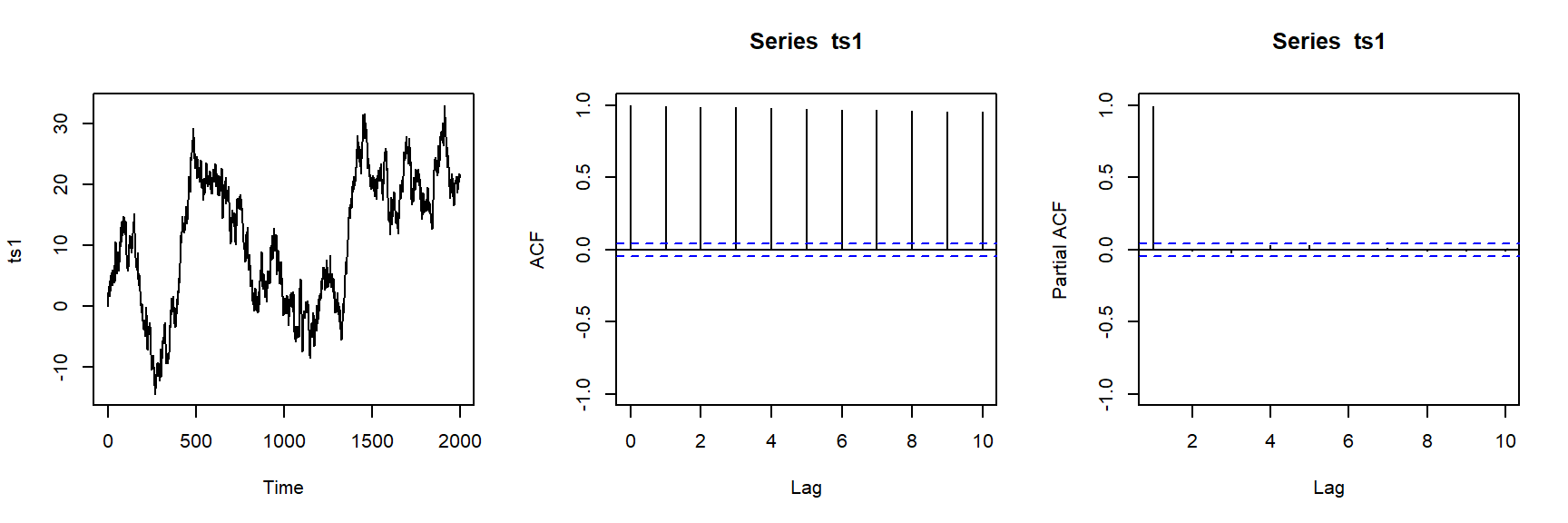plot.ts(diff(ts1))
acf(diff(ts1),ylim=c(-1,1),lag.max=10)
pacf(diff(ts1),ylim=c(-1,1),lag.max=10)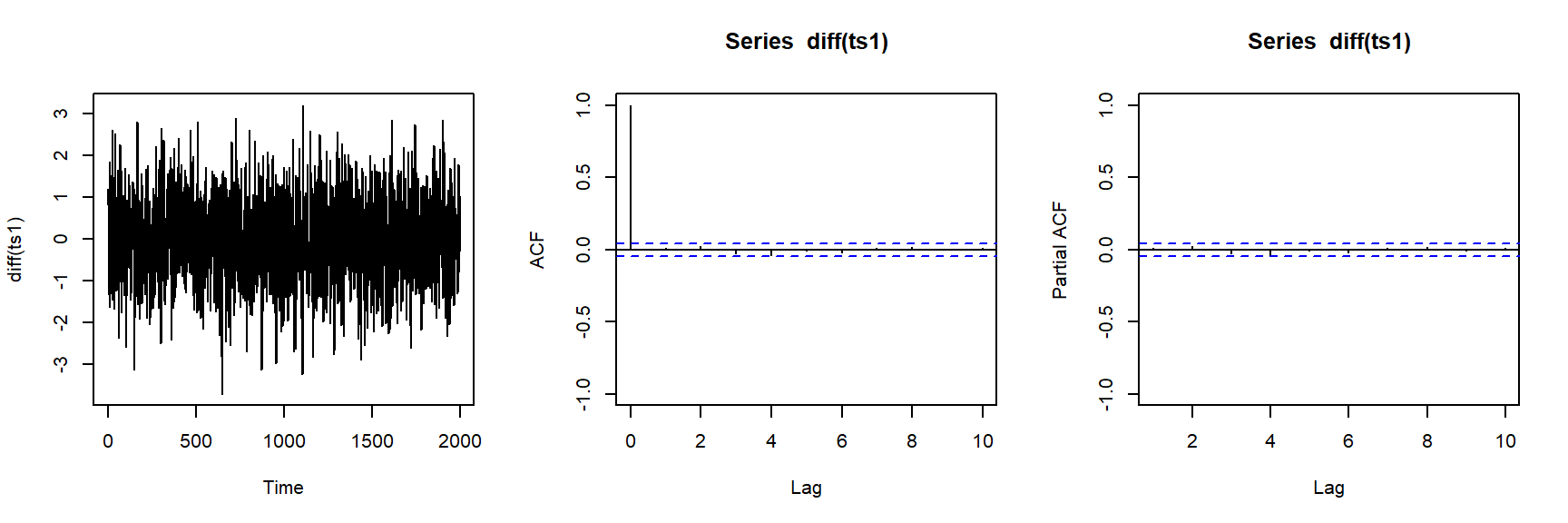## 2.3 ARIMA(0,1,1): Integrated Moving Average of order (1,1) or IMA(1,1)

\begin{align*} \tilde{Z}_t = \tilde{Z}_{t-1} + a_t - \theta_1 a_{t-1} \end{align*}

It is an appropriate model for a nonstationary time series data where

n=2000
ts1=arima.sim(n = n, list(order = c(0,1,1), ma = 0.5), sd = 1)
par(mfrow=c(1,3))
plot.ts(ts1)
acf(ts1,ylim=c(-1,1),lag.max=10)
pacf(ts1,ylim=c(-1,1),lag.max=10)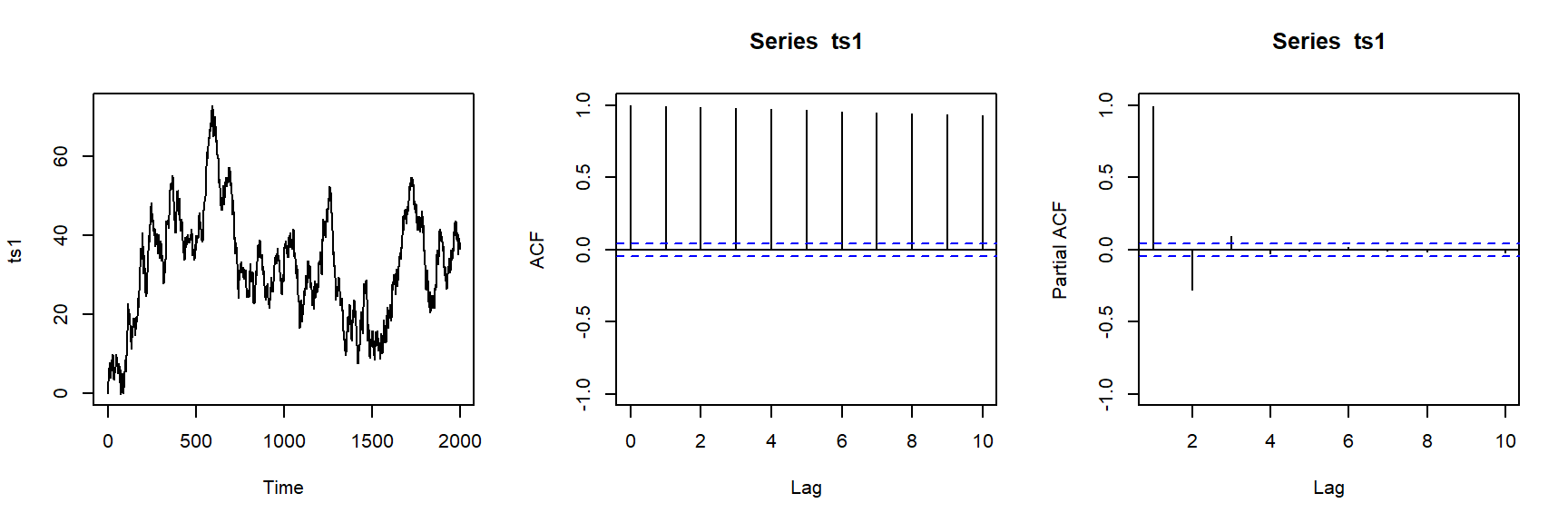plot.ts(diff(ts1))
acf(diff(ts1),ylim=c(-1,1),lag.max=10)
pacf(diff(ts1),ylim=c(-1,1),lag.max=10)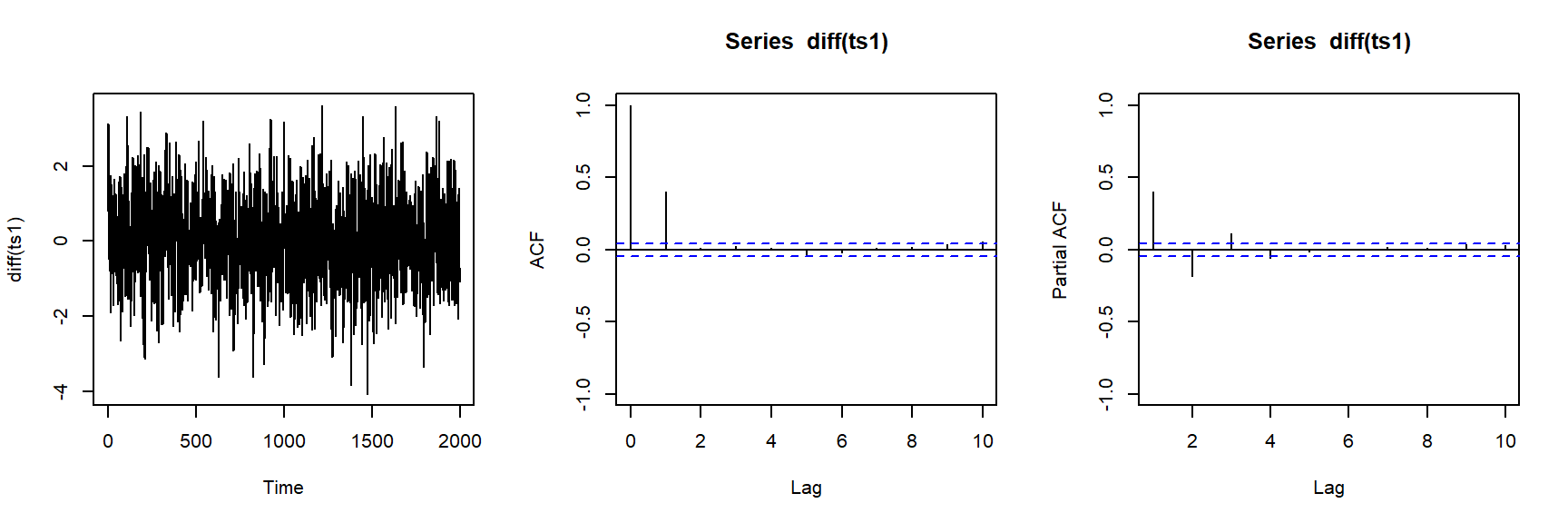## 2.4 Why called “integrated”?

Suppose $$Z_t$$ is an ARIMA(p,1,q). It follows that the 1st order difference $$W_t=Z_t-Z_{t-1}$$ is an ARMA(p,q). Since $$W_t=Z_t-Z_{t-1}$$, it is easy to show that \begin{align*} Z_t = W_t+W_{t-1}+W_{t-2}... \end{align*} To show the above equation, just think about $$Z_t-Z_{t-1}=(W_t+W_{t-1}+...)-(W_{t-1}+W_{t-2}+...)=W_t$$.

Since $$Z_t$$ is the summation of all the $$W_t$$ which an ARMA, $$Z_t$$ is an “integrated” ARMA, or ARIMA.